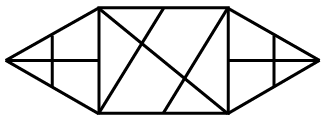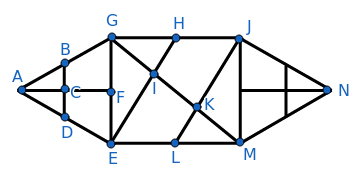Question 8

# How many triangles are present in the given figure?SolutionThe different triangles in figure AGE are ACB, ACD, ABD, AFG, AFE, AGE

Number of triangles in figure AGE = 6

Since figure AGE is similar to figure NJM, number of triangles in AGE is equal to number of triangles in NJM.

Number of triangles in figure NJM = 6

The remaining triangles in the given figure are GIH, GIE, GHE, MKJ, MKL, MJL, MIE, GJK, GJM, GEM

Number of remaining triangles = 8

$$\therefore\$$Number of triangles in the given figure = 6 + 6 + 8 = 22

Hence, the correct answer is Option C